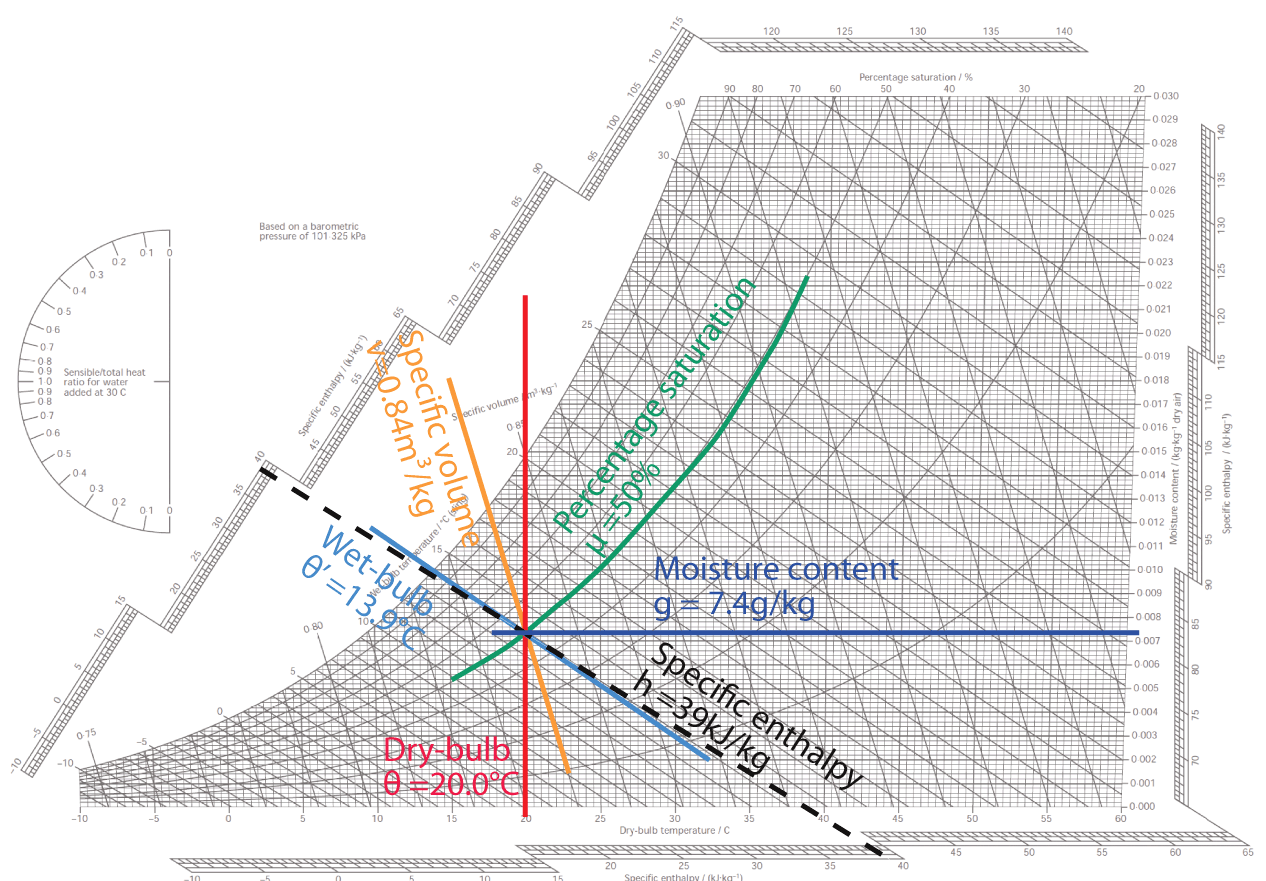## Translate

### How To Read a Psychrometric Chart? ( Clearly Explained Step by Step Process ).A psychrometric chart presents physical and thermal properties of moist air in a graphical form. It can be very helpful in roubleshooting greenhouse or livestock building environmental problems and in determining solutions. Understanding psychrometric charts helps visualization of environmental control concepts such as why heated air can hold more moisture, and conversely, how allowing moist air to cool will result in condensation. The objective of this fact sheet is to explain characteristics of moist air and how they are used in a psychrometric chart.

The first step in making a better measurement is to understand the parameter you wish to measure. What better way to begin to understand the various humidity parameters and their interrelations than to study the visual representation in a psychrometric chart.

Here are the easy 8 step framework designed to demystify the psychrometric chart:

Step 1:-
Locate the dry bulb temperature along the bottom axis and the associated vertical line for each temperature. This scale will be in degrees F or degrees C.

Step 2 :-
Locate the ‘humidity ratio’ along the right vertical axis. Humidity ratio units are grains of moisture per pound of dry air or grams of moisture per kilogram of dry air. The term ‘humidity ratio’ is sometimes used interchangeably with the term ‘mixing ratio’.

Step 3 :-
Locate the left most curved line. This is the saturation curve where the relative humidity is 100%. Other properties of the saturation curve include;
dew point temperature = dry bulb temperature;
wet bulb temperature = dry bulb temperature;
partial pressure of water vapor = saturation vapor pressure.

Step 4 :-
Locate the interior curved lines. Each of the lines represents a certain level of relative humidity in percent.

Step 5 :-
On the right side of the chart, locate the vertical line labeled ‘Dew Point’. Dew point is expressed as a temperature. The dew point lines traverse the chart as horizontal lines. What this tells you is that dew point does not change as the dry bulb temperature varies.

Step 6 :-
On the other side of the vertical line where you located dew point is the scale for vapor pressure expressed in inches of mercury or millibars. Vapor pressure lines also traverse the chart as horizontal lines.

Step 7 :-
Look at the information on all sides of the chart that is on the most extreme edge. These scales represent enthalpy expressed in BTU per pound of dry air. If you take a ruler, you could match the scales diagonally across the chart.

Step 8 :-
Look closely and find a second set of diagonal lines representing
web bulb temperature expressed as degrees F or degrees C.

Although the wet bulb lines are close to the enthalpy lines, they are not actually parallel to each other.

Tips and tricks for the psychrometric chart :-
As long as you have any 2 parameters where the lines will cross each other, you can determine all other parameters. For example, let’s say you know the dry bulb temperature is 70 degrees F and the
dew point is 50 degrees F. Take a ruler and draw a vertical line at the dry bulb temperature of 70 and draw a horizontal line where the dew point is 50. The point where these two lines intersect is known
as a ‘state point’. Plot the state point and you can also read the relative humidity, wet bulb, enthalpy, vapor pressure and humidity ratio. As another example, let’s say you know that the wet bulb temperature is 50 and the dry bulb temperature is 75. Plot the state point and read the rest of the parameters.

If you wanted to know the cooling effect of an evaporative cooler, plot the state point where the dry bulb temperature line and the wet bulb temperature line intersect. Or you could plot the point where the dry bulb temperature and the relative humidity intersect. Follow the wet bulb line all the way to the saturation curve. Then read the dry bulb temperature at that saturation point. The difference between the temperature at the original point and the final point is your effective cooling of the air. I suggest taking some time to get familiar with the chart. Knowing the psychrometric chart will help you understand the humidity parameters and their interrelations leading to a better measurement of
humidity.